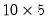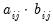Command Reference : Matrix Language : Matrix Commands and Functions

Matrix Commands and Functions
EViews provides a number of commands and functions that allow you to work with the contents of your matrix objects. These commands and functions may be divided into roughly four distinct types: (1) utility commands and functions, (2) element functions, (3) matrix algebra functions, and (4) descriptive statistics functions.
Utility Commands and Functions
The utility commands and functions provide support for creating, manipulating, and assigning values to your matrix objects. We have already seen a number of these commands and functions, including the @convert function and the stom command, both of which convert data from series and groups into vectors and matrices, as well as @vec, @vech, @rowextract, @columnextract, and matplace.
A random sampling of other useful commands and functions include:
matrix a = @ones(10, 5)
which creates amatrix of ones,
vector f = @getmaindiagonal(x)
which extracts the main diagonal from the square matrix X,
matrix g = @explode(sym01)
which creates a square matrix from the symmetric matrix object SYM01, and
matrix h1 = @resample(y)
matrix h2 = @permute(y)
which create matrices by randomly drawing (with replacement) from, and by permuting, the rows of Y.
A full listing of the matrix commands and functions is included in the matrix summary in  “Matrix Command and Function Summary”
Element Functions
EViews offers two types of functions that work with individual elements of a matrix object. First, most of the element functions that may be used in series expressions can used with matrix objects. When used with a matrix object, these functions will return a similar object whose elements are the values of a function evaluated at each element of the original.
For example, you may specify
matrix f = @log(y)
to compute the logarithm of each element of the matrix Y.
Similarly,
matrix tprob = @ctdist(x, df)
evaluates the cumulative distribution function value for the t-distribution for each element of the matrix X and places the results in the corresponding cells of the matrix TPROB. Note that DF may either be a scalar, or a matrix object of the same type and size as X.
(See “Basic Mathematical Functions”, “Special Functions”, “Trigonometric Functions”, and “Statistical Distribution Functions” for summaries of the various element functions.)
Second, EViews provides a set of element functions for performing element-by-element matrix multiplication, division, inversion, and exponentiation. For example, to obtain a matrix Z containing the inverse of every element in X, you may use:
matrix z = @einv(x)
Likewise, to compute the elementwise (Hadamard) product of the matrices A and B, you may use
matrix ab = @emult(a, b)
The (i,j)-th element of the matrix AB will contain the product of the corresponding elements of A and B:.
Matrix Algebra Functions
The matrix algebra functions allow you to perform common matrix algebra operations. Among other things, you can use these routines to compute eigenvalues, eigenvectors and determinants of matrices, to invert matrices, to solve linear systems of equations, and to perform singular value decompositions.
For example, to compute the inner product of two vectors A and B, you may use
scalar ip = @inner(a, b)
To compute the Cholesky factorization of a symmetric matrix G,
matrix cf = @cholesky(g)
The least squares coefficient vector for the data matrix X and vector Y may be computed as
vector b = @inverse(@inner(x))*@transpose(x)*y
or
vector b = @solvesystem(@inner(x), @transpose(x)*y)
A listing of the matrix algebra functions and commands is included in   “Matrix General Functions”
Descriptive Statistics Functions
The descriptive statistics functions compute summary statistics for the data in the matrix object. You can compute statistics such as the mean, median, minimum, maximum, and variance, over all of the elements in your matrix.
For example,
scalar xmean = @mean(xmat)
computes the mean taken over all of the non-missing elements of the matrix XMAT, and assigns the values to the scalar XMEAN. Similarly, the commands
scalar xquant95 = @quantile(xmat, .95)
computes the .95 quantile of the elements in XMAT.
Functions for computing descriptive statistics are discussed in “Descriptive Statistics”
In addition, there are functions for computing statistics for each column in a matrix.
vector xmeans = @cmean(xmat)
computes the mean for each column of XMAT and assigns the values to the vector XMEANS.
vector xmin = @cmin(xmat)
saves the column minimums in the vector XMIN. If, instead you wish to find the index of the minimum element for the column, you may use @cimin instead:
vector ximin = @cimin(xmat)
The column statistics are outlined in “Matrix Column Statistics Functions”.
Functions versus Commands
A function generally takes arguments, and always returns a result. Functions are easily identified by the initial “@” character in the function name.
There are two basic ways that you can use a function. First, you may assign the result to an EViews object. This object may then be used in other EViews expressions, providing you with access to the result in subsequent calculations. For example:
matrix y = @transpose(x)
stores the transpose of matrix X in the matrix Y. Since Y is a standard EViews matrix, it may be used in all of the usual expressions.
Second, you may use a function as part of a matrix expression. Since the function result is used in-line, it will not be assigned to a named object, and will not be available for further use. For example, the command:
scalar z = vec1*@inverse(v1+v2)*@transpose(vec1)
uses the results of the @inverse and @transpose functions in forming the scalar expression assigned to Z. These function results will not be available for subsequent computations.
By contrast, a command takes object names and expressions as arguments, and operates on the named objects. Commands do not return a value.
Commands, which do not have a leading “@” character, must be issued alone on a line, and may not be used as part of a matrix expression. For example, to convert a series X to a vector V1, you would enter:
stom(x, v1)
Because the command does not return any values, it may not be used in a matrix expression.
NA Handling
As noted above, most of the methods of moving data from series and groups into matrix objects will automatically drop observations containing missing values. It is still possible, however, to encounter matrices which contain missing values.
For example, the automatic NA removal may be overridden using the stomna command. Additionally, some of the element operators may generate missing values as a result of standard matrix operations. For example, taking element-by-element logarithms of a matrix using @log will generate NAs for all cells containing nonpositive values.
EViews follows two simple rules for handling matrices that contain NAs. For all operators, commands, and functions (with the exception of the descriptive statistics functions), EViews works with the full matrix object, processing NAs as required. For descriptive statistic functions, EViews automatically drops NAs when performing the calculation. These rules imply the following:
Matrix operators will generate NAs where appropriate. Adding together two matrices that contain NAs will yield a matrix containing NAs in the corresponding cells. Multiplying two matrices will result in a matrix containing NAs in the appropriate rows and columns.
All matrix algebra functions and commands will generate NAs, since these operations are undefined. For example, the Cholesky factorization of a matrix that contains NAs will contain NAs.
All utility functions and commands will work as before, with NAs treated like any other value. Copying the contents of a vector into a matrix using colplace will place the contents, including NAs, into the target matrix.
All of the matrix element functions will propagate NAs when appropriate. Taking the absolute value of a matrix will yield a matrix containing absolute values for non-missing cells and NAs for cells that contain NAs.
The descriptive statistics functions are based upon the non-missing subset of the elements in the matrix. You can always find out how many values were used in the computations by using the @obs or the @nas functions.